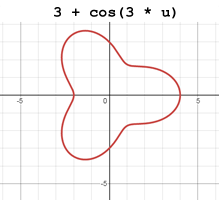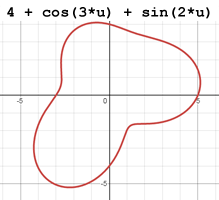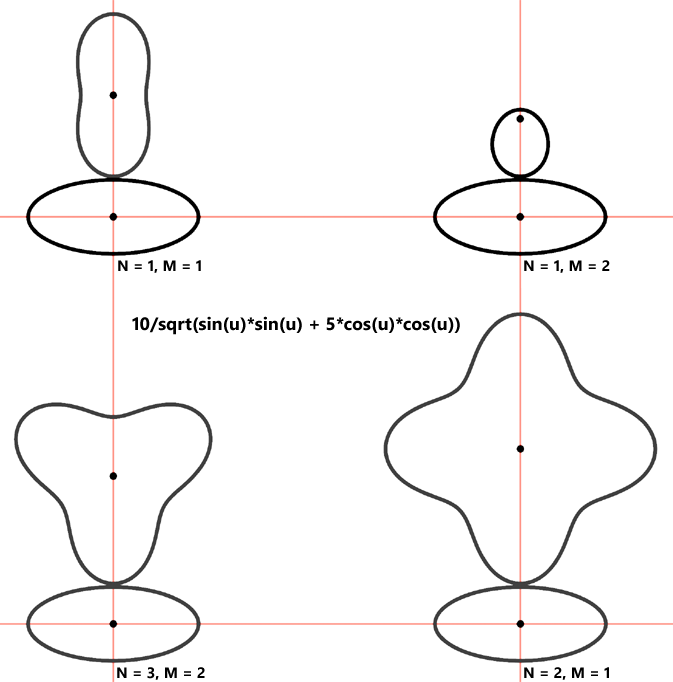Home  •  Tutorials  •  Calculators  •  3D Models  •  About  •  Contact

Non-Circular Gear Calculator

The following online calculator computes the raw outlines of two meshing non-circular gears based on the polar equation of Gear1, the desired Gear1-to-Gear2 pitch length ratio, and desired number of teeth.

Two meshing non-circular gears can conceptually be represented by two touching curves, called pitch or reference curves. The calculator allows the curve for Gear1 to be specified in the form of an arbitrary mathematical function f(u) in the polar coordinate system. The function must define a closed, non-intersecting and smooth curve. Below are two examples of functions which meet these requirements and are therefore suitable for non-circular gear design:The polar equation for Gear1 is to be entered in a syntax used by all programming languages: parentheses are required with mathematical functions, and the multiplication symbol (*) must not be omitted. Incorrect: sin 2u. Correct: sin(2*u).

The N and M parameters together specify the ratio of the lengths of Gear1's and Gear2's pitch curves. To put it differently, when Gear1 makes N full rotations, Gear2 makes M full rotations. The following diagram illustrates the effect of 4 different N/M combinations for this ellipse-defining function: 10 / sqrt( sin(u) * sin(u) + 5 * cos(u) * cos(u)).Note that the N and M values cannot be picked at random. They must reflect the periodicity of the main gear function. The ratio N/M times the number of periods of the function must be an integer. In the example above, the periodicity of the function is 2 and therefore (N=1, M=2) and (N=3, M=2) are valid combinations. If the periodicity were, say, 1, they would not be.

The Number of Teeth (Z) must be divisible by both N and M. The actual number of teeth in Gear1 is Z / N, and in Gear2 Z / M.

The Tooth Angle (α) specifies the slanting angle of the tooth flanks. It is 20° by default.

The Tooth Height Ratio specifies the height of each tooth relative to the distance between the teeth. It is 1 by default.

 Gear 1 Profile in the Form f(u) in Polar Coordinates Gear1-to-Gear2 pitch length ratio N / M / Number of Teeth, Z (Actual number of teeth: Z1 = Z / N, Z2 = Z / M) Tooth Angle, α (°) ° Tooth Height Ratio Blender 2.8+